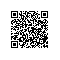# 好程序员web前端分享详细了解JavaScript函数

// 函数声明
function BindingIdentifier() {}

// 命名函数表达式
// (BindingIdentifier在函数外部是访问不到的)
(function BindingIdentifier() {});

// 匿名函数表达式
(function() {});

Function构造函数

new Function('x', 'y', 'return x ** y;');

// 另类匿名函数声明
export default function() {}

let object = {
propertyName: function() {},
};
let object = {
// (BindingIdentifier不能再函数外部调用)
propertyName: function BindingIdentifier() {},
};

let object = {
get propertyName() {},
set propertyName(value) {},
};

let object = {
propertyName() {},
["computedName"]() {},
get ["computedAccessorName"]() {},
set "computedAccessorName" {},
};

// 类声明
class C {
methodName() {}
["computedName"]() {}
get ["computedAccessorName"]() {}
set "computedAccessorName" {}
}

// 类表达式
let C = class {
methodName() {}
["computedName"]() {}
get ["computedAccessorName"]() {}
set "computedAccessorName" {}
};
...在定义静态方法时，同样可以使用。

// 类声明
class C {
static methodName() {}
static ["computedName"]() {}
static get ["computedAccessorName"]() {}
static set "computedAccessorName" {}
}

// 类表达式
let C = class {
static methodName() {}
static ["computedName"]() {}
static get ["computedAccessorName"]() {}
static set "computedAccessorName" {}
};

// 零参数, 赋值表达式
(() => 2 ** 2);

// 一个参数, 可以省略小括号, 赋值表达式
(x => x ** 2);

// 一个参数, 可以省略小括号, 函数体
(x => { return x ** 2; });

// 多个参数, 赋值表达式
((x, y) => x ** y);

let foo = x => x ** 2;

let object = {
propertyName: x => x ** 2
};
Generators

Generator函数的语法是在其他定义函数的方式上加点东西，但箭头函数和存取器方法除外。你可以使用和之前函数声明，函数表达式，函数定义甚至是构造函数等相似的方式。所有方法列举如下：

// Generator 声明
function *BindingIdentifer() {}

// 另类匿名 Generator 声明
export default function *() {}

// Generator 表达式
// (BindingIdentifier只能在函数内部调用)
(function *BindingIdentifier() {});

// 匿名 Generator 表达式
(function *() {});

// 方法定义
let object = {
*methodName() {},
*["computedName"]() {},
};

// 在类声明中定义方法
class C {
*methodName() {}
*["computedName"]() {}
}

// 在类声明中定义静态方法
class C {
static *methodName() {}
static *["computedName"]() {}
}

// 在类表达式中定义方法
let C = class {
*methodName() {}
*["computedName"]() {}
};

// 在类表达式中定义静态方法
let C = class {
static *methodName() {}
static *["computedName"]() {}
};
ES2017

// 异步函数声明
async function BindingIdentifier() { /**/ }

// 另类匿名异步函数声明
export default async function() { /**/ }

// 命名异步函数表达式
// (BindingIdentifier只能在函数内部调用)
(async function BindingIdentifier() {});

// 匿名异步函数表达式
(async function() {});

// 异步方法
let object = {
async methodName() {},
async ["computedName"]() {},
};

// 类声明中的异步方法
class C {
async methodName() {}
async ["computedName"]() {}
}

// 类声明中的静态异步方法
class C {
static async methodName() {}
static async ["computedName"]() {}
}

// 类表达式中的异步方法
let C = class {
async methodName() {}
async ["computedName"]() {}
};

// 类表达式中的静态异步方法
let C = class {
static async methodName() {}
static async ["computedName"]() {}
};

async 和 await并不是只局限在常规函数的声明或者表达式中，它们同样适用于箭头函数：

// 单一参数，赋值表达式
(async x => x ** 2);

// 单一参数，函数体
(async x => { return x ** 2; });

// 参数列表，赋值表达式
(async (x, y) => x ** y);

// 参数列表，函数体
(async (x, y) => { return x ** y; });

// 异步Generator声明
async function BindingIdentifier() { /*/ }

// 另类匿名异步Generator声明
export default async function *() {}

// 异步Generator表达式
// (BindingIdentifier只能在函数内部访问)
(async function *BindingIdentifier() {});

// 匿名异步Generator表达式
(async function *() {});

// 匿名异步Generator方法定义
let object = {
async *propertyName() {},
async *["computedName"]() {},
};

// 类声明中异步Generator原型方法定义
class C {
async *propertyName() {}
async *["computedName"]() {}
}

// 类表达式中异步Generator原型方法定义
let C = class {
async *propertyName() {}
async *["computedName"]() {}
};

// 类声明中异步Generator静态方法定义
class C {
static async *propertyName() {}
static async *["computedName"]() {}
}

// 类表达式中异步Generator静态方法定义
let C = class {
static async *propertyName() {}
static async *["computedName"]() {}
};使用钉钉扫一扫加入圈子
+ 订阅